# Sort by Second or Third character in a Column

This post will guide you how to sort text string by second or third character in a Column in Excel. You may be want to sort a string in a Cell by the Second or last character in excel, how to quickly do it? You can use the Excel Formula or Text to Column feature to solve this problem. The below will give you the detailed description.

## Excel sort by second character with Formula

To sort test string in a cell by its second character, you can use a combination of the RIGHT function and the LEN function to build a new Excel formula, you can refer to the following generic formula.

=RIGHT(Cell, LEN(Cell)-1)

This formula will remove the first character of the text string in Cell, then you can use the sort feature in Microsoft Excel to sort this column, and then the original column also will be sorted. Just do it following:

Assuming that you want to sort the range A1:A4 in Column A by second character.

1# select the cell B1 next to the cell A1 contains that you want to sort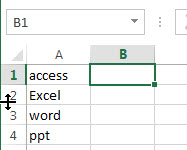2# type the following formula in the formula box of Cell B1, then press Enter key.

```=RIGHT(A1,LEN(A1)-1)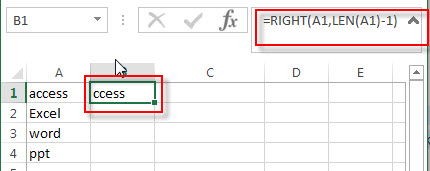```

3# select Cell B1, then drag the AutoFill Handle down to the cell B2:B4 to apply this formula. You will see that the first character will be deleted.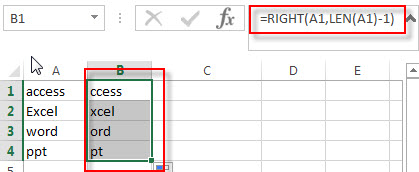4# select the range B1:B4, on the DATA tab, click sort A to Z command under Sort & Filter group.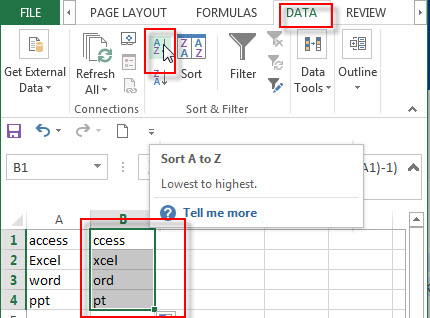5# choose Expand the selection radio button in the Sort Warning window. Then click Sort button.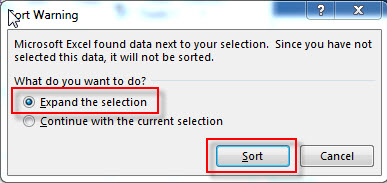6# you will see that the column A and B are sorted by the second character.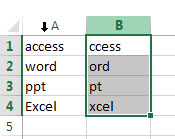7# you can remove the column B now.

## Excel sort by second character with Text to Columns

If the text strings are joined by the delimited character, and you want sort text string by the middle characters, then you can use the Text to Columns feature to achieve the result. Or you can use the MID function to extract the middle characters, then use the Sort feature in Excel to sort them.

Just refer to the following steps:

1# select the range of cells contain the text string that you want to sort.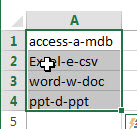2# on the DATA tab, click Text to Column command, the Convert Text to Columns Wizard window will appear.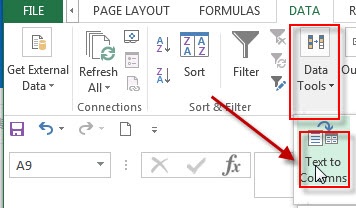3# choose Delimited radio button in the first step window, click Next button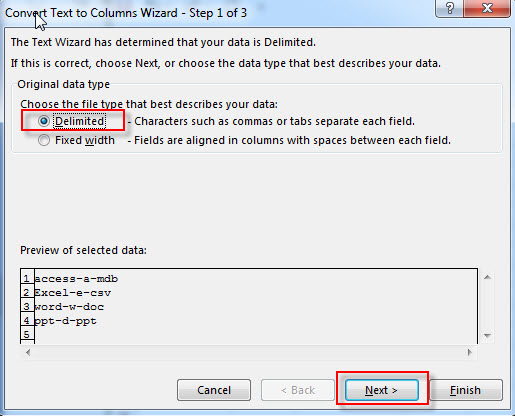4# type the delimited character which is used to join the text string in Cell, click Next button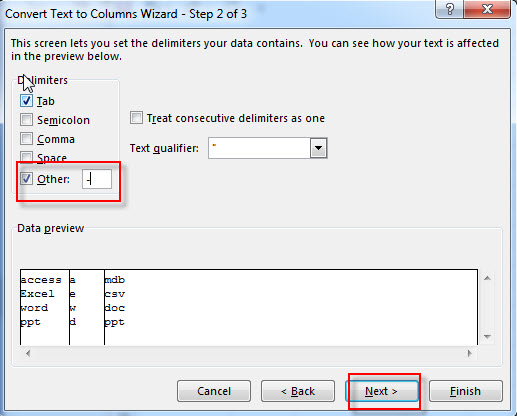5# choose the destination cell reference, then click Finish button.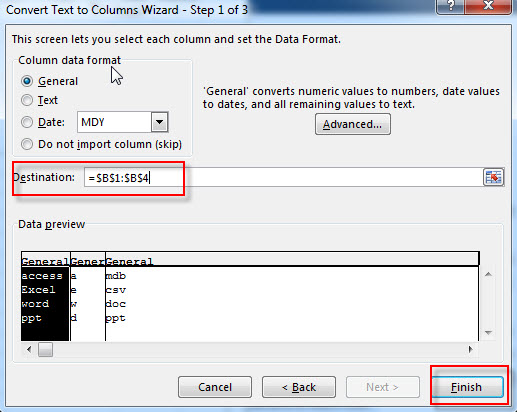6# you will see that the text string is split into three columns, and then you can select the column that contain the middle characters, click Sort A to Z command under DATA tab, the columns are sorted by the middle characters in each cell.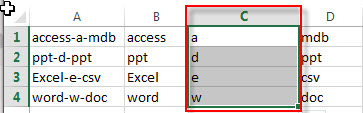This method is only available for the text string that joined by the specific character or delimiter. So if the text string in Cell do not have any common delimiter, then you can use the MID function to extract the middle characters, then following the Step5 to sort the text string. You can use the following generic formula:

`=MID(A1,FIND("-",A1)+1,1)`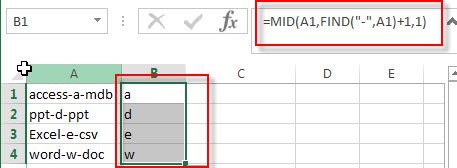### Related Formulas

• Sort Names by Middle Name in Excel
Assuming that you have a list of names in your worksheet and you would like to alphabetize by middle name. You can create an excel formula based on the IF function, the ISERR function, the FIND function, and the MID function.…
• Split Multiple Lines from a Cell into Rows
If you have multiple lines in a cell and each line is separated by line break character or press “alt + enter” key while you entering the text string into cells, and you need to extract the multiple lines into the separated rows or columns, you can use a combination with the TRIM function, the MID function, the SUBSTITUTE function, the REPT function, the LEN function to create a complex excel formula..…
• Extract nth word from text string
If you want to extract the nth word from a text string in a single cell, you can create an excel formula based on the TRIM function, the MID function, the SUBSTITUTE function, the REPT function and the LEN function..…
• Get last word from text string
If you want to get the last word from a text string, you can create an excel formula based on the RIGHT function, the LEN function, the FIND function and the SUBSTITUTE function..…
• Extract word that starting with a specific character
Assuming that you have a text string that contains email address in Cell B1, and if you want to extract word that begins with a specific character “@” sign, you can use a combination with the TRIM function, the LEFT function, the SUBSTITUTE function, the MID function, the FIND function, the LEN function and the REPT function to create an excel formula.…

### Related Functions

• Excel RIGHT function
The Excel RIGHT function returns a substring (a specified number of the characters) from a text string, starting from the rightmost character.The syntax of the RIGHT function is as below:= RIGHT (text,[num_chars])…
• Excel LEN function
The Excel LEN function returns the length of a text string (the number of characters in a text string).The syntax of the LEN function is as below:= LEN(text)…
• Excel FIND function
The Excel FIND function returns the position of the first text string (sub string) within another text string.The syntax of the FIND function is as below:= FIND(find_text, within_text,[start_num])…
• Excel MID function
The Excel MID function returns a substring from a text string at the position that you specify.The syntax of the MID function is as below:= MID (text, start_num, num_chars)….

Sidebar